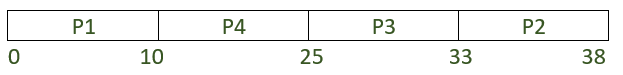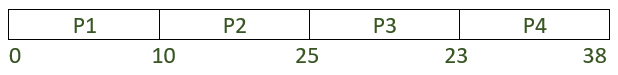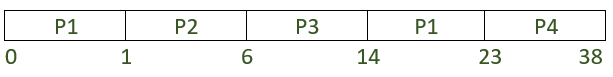# Difference between SJF and LJF CPU scheduling algorithms

Shortest Job First:
The shortest job first (SJF) algorithm is a CPU scheduling algorithm designed to reorder the jobs so that the process having the smallest burst time is chosen for the next execution. It is used to reduce the average waiting time for other processes waiting for execution. This may be preemptive or non-preemptive. Its preemptive version is called Shortest Remaining Time First (SRTF).

When a job comes in, it is inserted in the ready queue based on its burst time. SJF minimizes the average wait time because it gives service to less burst time processes before it gives service to processes with larger burst time.

The major advantage of using this algorithm is that, it increases the average turn-around time and average waiting time thus increasing the effectiveness of the system.

While it minimizes average wait time, it may punish processes with higher burst time. If shorter execution time processes are in the ready list, then processes with large burst times tend to be left in the ready list while small processes receive service. It may happen in extreme cases that always short execution time processes will be served and large execution time processes will wait indefinitely. This starvation of longer execution time processes is the limitation of this algorithm.

Longest Job First:
Longest job first (LJF) on the other hand is the exact opposite of SJF. It’s designed to reorder the jobs so that the process having the largest burst time is chosen for the next execution. It a type of non-preemptive scheduling algorithm where once a process starts its execution, it cannot be interrupted in between its processing and any other process can be executed only after the assigned process has completed its processing and has been terminated. When a job comes in, it is inserted in the ready queue based on its burst time. This may also be preemptive or non-preemptive.
Its preemptive version is called Longest Remaining Time First (LRTF).

The major disadvantage of this algorithm is that it might lead to starvation of processes. Convoy effect is another problem and leads to reduced throughput. Therefore this algorithm is seldom used.

Consider the following example:

Let’s try and solve this problem using both SJF and LJF to do a comparative study.

1. Longest Job First:
The Gantt chart will look like:```Average waiting time (AWT),
= ((0-0) + (33-1) + (25-2) + (10-2)) / 4
= 63/4
= 15.75

Average turnaround time (ATAT),
= ((10-0) + (38-1) + (33-2) + (25-2))/4
= 101/4
= 25.25 ```

2.(a). Shortest Job First (Non-Preemptive):
The Gantt chart will look like:```Average waiting time (AWT),
= ((0-0) + (10-1) + (15-2) + (23-2)) / 4
= 43 / 4
= 10.75

Average turnaround time (ATAT),
= ((10-0) + (15-1) + (23-2) + (38-2)) / 4
= 81 / 4
= 20.25 ```

2.(b). Shortest Job First (Preemptive):
The Gantt chart will look like:```Average waiting time (AWT),
= ((0-0) + (1-1) + (6-2) + (23-2)) / 4
= 25 / 4
= 6.25

Average turnaround time (ATAT),
= ((23-0) + (6-1) + (14-2) + (38-2)) / 4
= 76 / 4
= 19 ```

From the above example it is clear that SJF algorithm is more efficient than LJF algorithm.